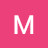# Silvaco TCAD ATLAS tutorial 6. Design of DGMOSFET. How to find out SS, Vth, Ion/Ioff ratio and DIBL.

• 🎬 Video
• ℹ️ DescriptionUCbl4Xihpgcwt_niSXRHi6YA

Design of Double Gate Mosfet (DGMOSFET).
Design and Characterization of Double Gate MOSFET using Silvaco TCAD tool.
Comparison between DGMOSFET, SOI and Bulk MOSFET.
How to extract different parameters such as Subthreshold Slope (SS), Threshold Voltage (Vth), Ion/Ioff ratio and Drain Induces Barrier Lowering (DIBL) using Silvaco Tool.

Hello to all
Thank you so much for your support and love.
I am giving silvaco Tcad code explaining fundamental of nanotechnology devices for rupees 15,000 only (more than 15 codes). The beginner in this field can avail this opportunity to become the masters.

For one to one guidance from basic to advance level coding and theory session, the charges are rupees 5000 to 15000.

The list of codes that I will be providing incudes:
Design of Bulk MOSFET, SOI MOSFET (PD & FD), DGMOSFET, TFET, Nanowire FET, Nanowire FET for Gas sensing, Triple Metal Gate Vertical TFET, JLTFET Biosensor, CPTFET Biosensor Ferroelectric FET, Organic FET, GaN nanowire, AlGaN/GaN HEMT, FinFet, Dual Source VTFET.
Simulation PPT - 450 slides + Modeling - 62 slides (Both in rupees 7,000 only).
Offer is valid up to 31st march 2021.

For Indian user

For user outside India

One more thing if anyone looking for B.tech project, M.tech assistance, Ph.D. assistance and Thesis writing help, we are providing this service in India. We offer M.tech, Ph.D. Support with Journal publication, Synopsis, Thesis writing, and Research Proposal. M.tech, Ph.D. Publishing Services includes SCI, Scopus, IEEE, Elsevier, & Springer indexed journals, Citation Services and Patent Services.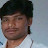you have to develop a 3D 14nm gate length Tri-Gate FinFET in Atlas with the following specifications:

1. Length of source/drain = 10 nm

2. Height of source/drain = 20 nm

3. Width of source/drain = 20 nm

4. Doping of source/drain = n-type, 1 x 10^18

5. Doping of substrate and channel = p-type, 2 x 10^15

6. Tbox = 50 nm, Tsub = 30 nm

7. Gate Work function = 4.6eV

8. Thickness of SiO2 oxide layer = 2 nm.

Author — Rajesh nathji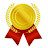Can u make Video on flash memory...mainly address to threshold value

Author — Mamatha Gollavilli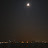Hello, tks for the videos.
Do you have any DRAM devices or library with the parameters (geometry, doping profile ...) that is available for academic use ?

Author — Nguyen Hoang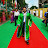Sir, the extraction is not occuring. It says no such file in the directory and initialisation has not been performed. I am using example soiex02. What to do??

Author — Rahul Paul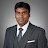Nice information, thank you very much.

Author — Murugapandiyan P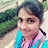Great video!! what is the nw_gc_igdl.log
file that you've used?!

Author — Akshaya Anand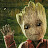Sir can you please tell how to simulate bandgap and electric field models for 2-D structured Dual Gate Mosfet

Author — Mayuresh Joshi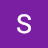Hii sir.. in the beginning u have shown device dimensions as Length = 500 nm .. but while writing x.mesh commands u took length as only 250nm. Infact all dimensions are different

Author — Sana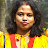sir, extract DIBL code is not working, can you help sir ?

Author — Sudeshna Mondal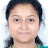Very informative, can you show for Double gate FINFET also. Thank you.

Author — Salini Singh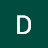When execution this code, I am not getting proper output file. Plz help sir

Author — Diganta Das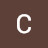Hi Sir. It was a great video, thanks. Nervetheless i have a question for you. Did you use the model that you present in video?
Because the dimensions doesn't agree with the 2-D model in tonyplot, you have been construct using parameters that are half part of the model that you present, that is what i think, maybe i made a mistake.

And finally the results are different to me, despite i copy your code. Thanks for the answer

Author — Carlos Castle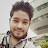Why did you take "y intercept=-7" for calculating threshold voltage?

Author — Shirazush Salekin Chowdhury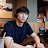Hello sir. Thank you for nice videos. I've got two problem. Can I get some advice for these issues?

1.Tonyplot of "nw_gc_igd1.log"
In my plot, first point (0V, 0A)and last point(1V, 0.1mA) are connected together.
But, I can see your plot is not like me. (6:56)

2. Error message
Below is the error message I got.

Unrecognized line in set file "nw_gc_idg.log":v ATLAS
Do you wish to suppress warnings?

What can I do for this?

Double gate
go atlas
mesh space.mult=1.0
#
x.mesh loc=0.00 spac=0.01
x.mesh loc=0.005 spac=0.01
x.mesh loc=0.10 spac=0.0001
x.mesh loc=0.15 spac=0.0001
x.mesh loc=0.20 spac=0.01
x.mesh loc=0.25 spac=0.0001
#
y.mesh loc=0.00 spac=0.001
y.mesh loc=0.002 spac=0.001
y.mesh loc=0.005 spac=0.001
y.mesh loc=0.015 spac=0.001
y.mesh loc=0.018 spac=0.001
y.mesh loc=0.020 spac=0.001

#
region num=1 material=Air x.min=0 y.min=0
region num=2 material=SiO2 x.min=0.1 x.max=0.15 y.min=0.002 y.max=0.005
region num=3 material=SiO2 x.min=0.1 x.max=0.15 y.min=0.015 y.max=0.018
region num=4 material=silicon x.min=0 x.max=0.1 y.min=0.005 y.max=0.015
region num=5 material=silicon x.min=0.1 x.max=0.15 y.min=0.005 y.max=0.015
region num=6 material=silicon x.min=0.15 x.max=0.25 y.min=0.005 y.max=0.015
#
electrode name=gate x.min=0.1 x.max=0.15 y.min=0.0 y.max=0.002
electrode name=gate2 x.min=0.1 x.max=0.15 y.min=0.18 y.max=0.20
electrode name=source x.min=0 x.max=0.05 y.min=0.003 y.max=0.005
electrode name=drain x.min=0.2 x.max=0.25 y.min=0.003 y.max=0.005
#
doping uniform concentration=1e18 p.type region=5
doping uniform concentration=1e20 n.type region=4
doping uniform concentration=1e20 n.type region=6
#
interf qf=3e10 y.max=0.005
#
contact name=gate workfun=4.6
contact name=gate2 workfun=4.6 common=gate
contact name=source
contact name=drain
#
#
models conmob srh auger bgn fldmob print
#
output val.band con.band qfn qfp e.field j.electron j.hole j.conduction j.total ex.field ey.field flowline e.mobility h.mobility qss e.temp h.temp j.disp
#
method newton trap
#
solve init
solve prev
solve vdrain=0
solve vdrain=0.01
solve vdrain=0.02
solve vdrain=0.03
solve vdrain=0.04
solve vdrain=0.05
log outf=nw_gc_igd1.log
solve name=gate vgate=0 vstep=0.1 vfinal=1
tonyplot nw_gc_igd1.log
save outf=nw_gc_igd_eq.str
tonyplot nw_gc_igd_eq.str

#extract init infile="nw_gc_igd1.log"
extract name="vt1" x.val from curve ((v."gate"), log10(abs(i."drain"))) where y.val=-7

solve init
solve prev
solve vdrain=0
solve vdrain=0.05
solve vdrain=0.1
solve vdrain=0.2
solve vdrain=0.4
solve vdrain=0.6
solve vdrain=0.8
solve vdrain=1
log outf=nw_gc_igd.log

solve ac freq=1e16 name=gate vgate=0 vstep=0.05 vfinal=1
#extract init infile="nw_gc_igd.log"

extract name="vt2" x.val from curve ((v."gate"), log10(abs(i."drain"))) where y.val=-7
save outf=nw_gc_igd_eq.str
tonyplot nw_gc_igd.log

tonyplot -overlay nw_gc_igd1.log nw_gc_igd.log -set nw_gc_igd.log

extract name="dibl" (\$"vt1"-\$"vt2")/(1-0.05)
#extract init infile="nw_gc_igd.log"
extract name="subvt" 1.0/slope(maxslope(curve(v."gate",
extract name="Ioff_n" y.val from curve(v."gate", i."drain") where x.val=0
extract name="Ion_n" y.val from curve(v."gate", i."drain") where x.val=1
extract name="Ion_Ioff" \$Ion_n/\$Ioff_n

extract name="gm" curve (v."gate", dydx(v."gate", i."drain")) outf="gm.dat"

quit

Author — 홍인표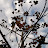Why interface oxide charges are not defined for the second gate?

Author — Salini